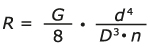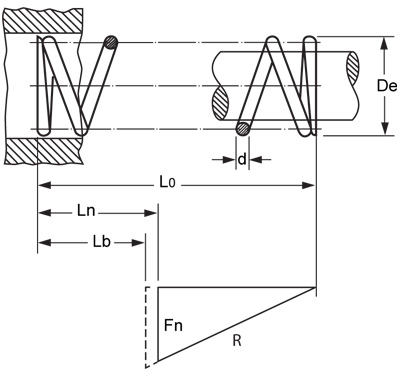# How do you calculate the spring constant of a compression spring?

The spring constant is always designated as N/mm (Newton per millimetre) 1N = approx. 0.1kg

The spring constant is calculated according to the following formula:R = Spring constant
G = Modulus of shear N/mm2
d4 = wire thickness to the power of 4
D3 = Mean diameter to the power of 3
n = active coils

The spring constant describes how much the force in Newtons is increased per mm the spring is compressed.
The spring constant is linear for a cylindrical compression spring.

The spring constant is the result of the combination of wire thickness, diameter, number of active coils and free length.
The drawing below shows the increase in force Fn as a result of R.## Internet Explorer Incompatibility

This website unfortunately does not work well with Internet Explorer. We recommend using one of the following free browsers: